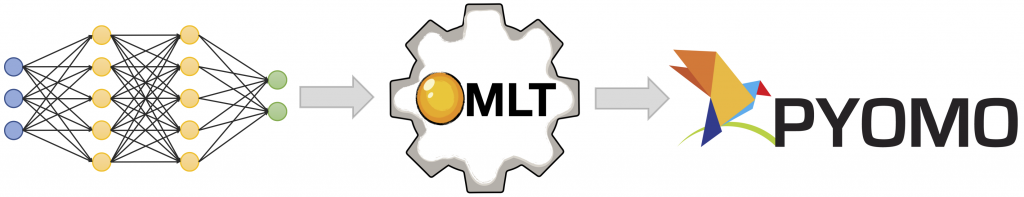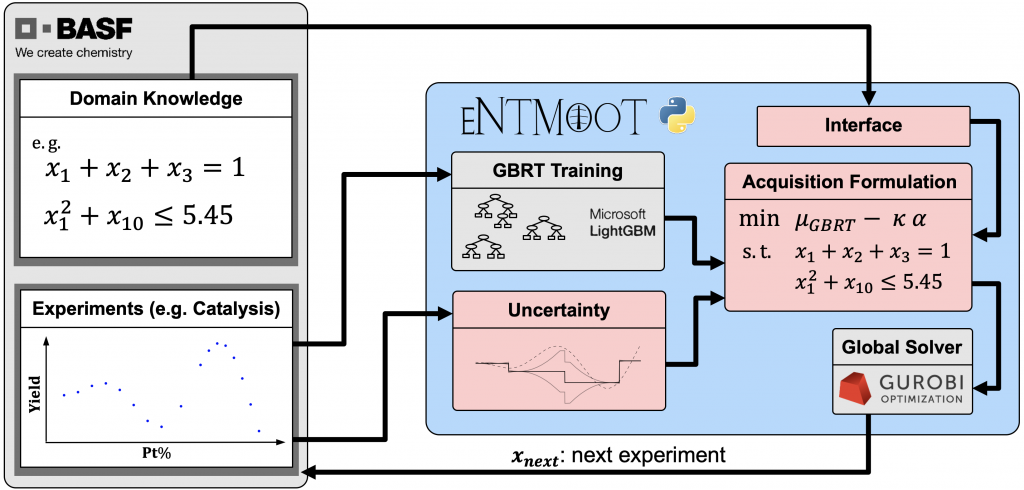# Optimization over machine learning surrogates

## Summary

Many interesting optimization problems combine both data-driven surrogates and mechanistic models. For example, consider a supply chain application where a transshipment model, i.e. a linear program, represents the flow of material and a data-driven model, e.g. a neural network, represents demand. The resulting optimization problem requires simultaneously optimizing over both (i) equations representing the supply chain and (ii) the already-trained neural network.

Different than classic machine learning, the neural network weights and biases are now held constant. Instead, we use the inputs x and outputs y of the neural network as decision variables:Other instances of this problem arise in (i) black-box optimization over tree-based models with input constraints, (ii) engineering applications where machine learning models replace complicated constraints or act as surrogates, or (iii) maximizing a neural acquisition function.

## What contributions have we made?

• Optimization over gradient-boosted trees with additional constraints (Mistry et al., 2021). Our custom branch-and-bound strategy explicitly uses the patterns already in the trees.
• Partition-based formulations (Kronqvist et al., 2021, Tsay et al., 2021). Many optimization formulations are written with either a big-M or a convex hull formulation. Prior literature often observes that the big-M formulation is too light and the convex hull formulation is too heavy. Our Goldilocks-style solution makes the optimization formulation just right for the application.

• Tree ensemble kernels for Bayesian optimization (Thebelt et al., 2022). We use the kernel interpretation of tree ensembles as a Gaussian Process prior to obtain model variance estimates and develop an optimization formulation for the acquisition function.

## Software

### OMLT: Optimization & machine learning toolkitOMLT is a Python package for representing machine learning models (neural networks and gradient-boosted trees) within the Pyomo optimization environment (Ceccon, Jalving, et al., 2022). OMLT was awarded the 2022 COIN-OR Cup as the best contribution to open-source operations research software development.

Here is an introduction to OMLT and a discussion of switching optimization formulations in OMLT:

### ENTMOOT: Multiobjective black-box optimization using gradient-boosted trees

In collaboration with researchers from BASF, Alexander Thebelt developed ENTMOOT to handle tree-based models in Bayesian optimization (Thebelt et al., 2021). We use Gurobi as the underlying solver to optimize over both the gradient-boosted trees and the input constraints.Here is an introduction to ENTMOOT and a discussion of extending ENTMOOT to the multi-objective setting (Thebelt et al., 2022):